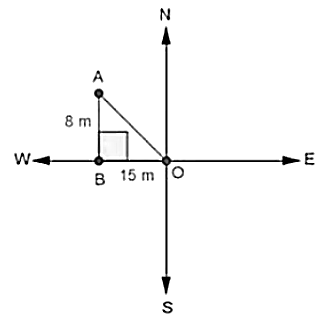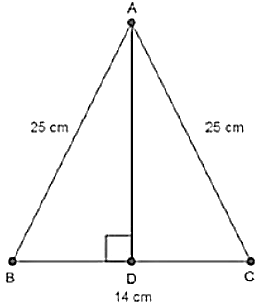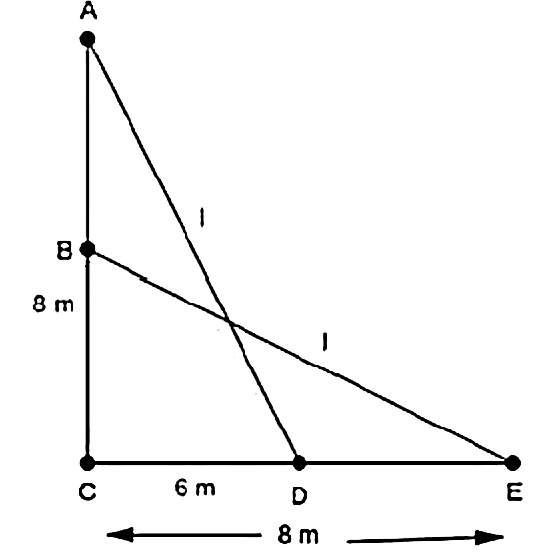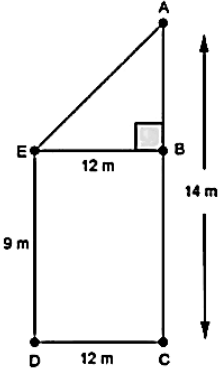# RD Sharma Class 10 Ex 4.7 Solutions Chapter 4 Triangles

In this chapter, we provide RD Sharma Class 10 Ex 4.7 Solutions Chapter 4 Triangles for English medium students, Which will very helpful for every student in their exams. Students can download the latest RD Sharma Class 10 Ex 4.7 Solutions Chapter 4 Triangles pdf, Now you will get step by step solution to each question.

# Chapter 4: Triangles Exercise – 4.7

### Question: 1

If the sides of a triangle are 3 cm, 4 cm, and 6 cm long, determine whether the triangle is a right-angled triangle.

### Solution:

We have,

Sides of triangle

AB = 3 cm

BC = 4 cm

AC = 6 cm

∴  AB2 = 32 = 9

BC= 42 = 16

AC2 = 62 = 36

Since, AB2 + BC2 ≠ AC2

Then, by converse of Pythagoras theorem, triangle is not a right triangle.

### Question: 2

The sides of certain triangles are given below. Determine which of them right triangles are.

(i) a = 7 cm, b = 24 cm and c = 25 cm

(ii) a = 9 cm, b = 16 cm and c = 18 cm

(iii) a = 1.6 cm, b = 3.8 cm and c = 4 cm

(iv) a = 8 cm, b = 10 cm and c = 6 cm

### Solution:

We have,

a = 7 cm, b = 24 cm and c = 25 cm

∴ a2 = 49, b2 = 576 and c2 = 625

Since, a2 + b2 = 49 + 576 = 625 = C2

Then, by converse of Pythagoras theorem, given triangle is a right triangle.

We have,

a = 9 cm, b = 16 cm and c = 18 cm

∴ a2 = 81, b2 = 256 and c2 = 324

Since, a2 + b2 = 81 + 256 = 337 ≠ c2

Then, by converse of Pythagoras theorem, given triangle is not a right triangle.

We have,

a = 1.6 cm, b = 3.8 cm and C = 4 cm

∴ a2 = 64, b2 = 100 and c2 = 36

Since, a2 + c2 = 64 + 36 = 100 = b2

Then, by converse of Pythagoras theorem, given triangle is a light triangle.

### Question: 3

A man goes 15 metres due west and then 8 metres due north. How far is lie from the starting point?

### Solution:Let the starting point of the man be O and final point be A.

∴ In ∆ABO, by Pythagoras theorem AO2 = AB2 + BO2

⟹ AO2 = 82 + 152

⟹ AO2 = 64 + 225 = 289

⟹ AO =  √289 = 17m

∴  He is 17m far from the starting point.

### Question: 4

A ladder 17 m long reaches a window of a building 15 m above the ground. Find the distance of the foot of the ladder from the building.

### Solution:In ∆ABC, by Pythagoras theorem

AB2 + BC2 = AC2

⟹ 152 + BC2 = 172

⟹ 225 + BC2 = 172

⟹ BC2 = 289 – 225

⟹ BC2 = 64

⟹ BC = 8 m

∴ Distance of the foot of the ladder from building = 8 m

### Question: 5

Two poles of heights 6 m and 11 m stand on a plane ground. If the distance between their feet is 12 m, find the distance between their tops.

### Solution:

Let CD and AB be the poles of height 11 and 6 in.Therefore CP = 11 – 6 = 5 m

From the figure we may observe that AP = 12m

In triangle APC, by applying Pythagoras theorem

Ap2 + PC2 = AC2

122 + 52 = AC2

AC2 = 144 + 25 = 169

AC = 13

Therefore distance between their tops = 13 m.

### Question: 6

In an isosceles triangle ABC. AB = AC = 25 cm, BC = 14 cm. Calculate the altitude from A on BC.

### Solution:

We have

AB = AC = 25 cm and BC = 14In ∆ABD and ∆ACD

AB = AC                                [Each = 25 cm]

Then, ∆ABD ≅ ∆ACD      [By RHS condition]

∴ BD = CD = 7 cm              [By c.p.c.t]

⟹ AD2 + 72 = 252

⟹ AD2 = 625 – 49 = 576

⟹ AD = √576 = 24 cm

### Question: 7

The foot of a ladder is 6 m away from a wall and its top reaches a window 8 m above the ground. If the ladder is shifted in such a way that its foot is 8 m away from the wall, to what height does its tip reach?

### Solution:Let length of ladder be AD = BE = l m

In ∆ACD, by Pythagoras theorem

⟹ l2 = 82 + 62 … (i)

In ∆BCE, by pythagoras theorem

BE2 = BC2 + CE2

⟹ l2 = BC2 + 82 … (ii)

Compare (i) and (ii)

BC2 + 82 = 82 + 62

⟹ BC2 + 62

⟹ BC = 6 m

### Question: 8

Two poles of height 9 in and 14 m stand on a plane ground. If the distance between their feet is 12 in, find the distance between their tops.

### Solution:We have,

AC = 14 m. DC = 12m and ED = BC = 9 m

Construction: Draw EB ⊥ AC

∴ AB = AC –  BC = 14 — 9 = 5 m

And, EB = DC = 12m

In ∆ABE, by Pythagoras theorem,

AE2 = AB2 + BE2

⟹ AE2 = 52 + 122

⟹ AE2 = 25 + 144 = 169

⟹ AE= √169 = 13 m

∴  Distance between their tops = 13 m

### Question: 9

Using Pythagoras theorem determine the length of AD in terms of b and c shown in Fig. 4.219

### Solution:

We have,

In ∆BAC, by Pythagoras theorem

BC2 = AB2 + AC2

⟹ BC2 = c2 + b2

In ∆ABD and ∆CBA

∠B = ∠B                      [Common]

Then, ∆ABD ͏~ ∆CBA  [By AA similarity]

[Corresponding parts of similar ∆ are proportional]

### Question: 10

A triangle has sides 5 cm, 12 cm and 13 cm. Find the length to one decimal place, of the perpendicular from the opposite vertex to the side whose length is 13 cm.

### Solution:Let, AB = 5cm, BC = 12 cm and AC = 13 cm. Then, AC2 = AB2 + BC2. This proves that ∆ABC is a fight triangle. right angles at B. Let BD be the length of perpendicular from B on AC.

= 30 cm2

All Chapter RD Sharma Solutions For Class10 Maths

I think you got complete solutions for this chapter. If You have any queries regarding this chapter, please comment on the below section our subject teacher will answer you. We tried our best to give complete solutions so you got good marks in your exam.